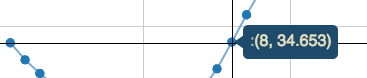# (x,y) tooltips without colon on Bokeh

I would like to create a tooltip that simply shows (x,y) values on it. I tried to do so as follows, but it still shows a colon. How can I remove it?

plot.line(‘x’, ‘y’, source=my_data,)

plot.circle(‘x’, ‘y’, size=8, alpha=1, color=color, source=to_plot)

hover = plot.select(dict(type=HoverTool))

hover.tooltips = [

(’’, “(@x, @y)”),

]Hi,···

On Thu, Feb 26, 2015 at 7:47 PM, Josh Wasserstein [email protected] wrote:

I would like to create a tooltip that simply shows (x,y) values on it. I tried to do so as follows, but it still shows a colon. How can I remove it?

plot.line(‘x’, ‘y’, source=my_data,)

plot.circle(‘x’, ‘y’, size=8, alpha=1, color=color, source=to_plot)

hover = plot.select(dict(type=HoverTool))

hover.tooltips = [

(’’, “(@x, @y)”),

]

since bokeh 0.8.1 you can pass a string to hover.tooltips instead of a list of tuples, so hover.tooltips = “(@x, @y)” will do what’s expected. You can use HTML tags (and CSS styling) as well, e.g. hover.tooltips = “”"(@x, @y""" (@x and @y will be escaped).

Mateusz

You received this message because you are subscribed to the Google Groups “Bokeh Discussion - Public” group.

To unsubscribe from this group and stop receiving emails from it, send an email to [email protected].

To post to this group, send email to [email protected].

That worked! Thanks Mateusz.

Josh···

On Thu, Feb 26, 2015 at 4:33 PM, Mateusz Paprocki [email protected] wrote:

Hi,

You received this message because you are subscribed to the Google Groups “Bokeh Discussion - Public” group.

To unsubscribe from this group and stop receiving emails from it, send an email to [email protected]ontinuum.io.

To post to this group, send email to [email protected].

To view this discussion on the web visit https://groups.google.com/a/continuum.io/d/msgid/bokeh/CANFzp8hXjEMfyFCZsrpmet8PCAp5u3TNfpGOk_aMhnceFi9unA%40mail.gmail.com.

On Thu, Feb 26, 2015 at 7:47 PM, Josh Wasserstein [email protected] wrote:

I would like to create a tooltip that simply shows (x,y) values on it. I tried to do so as follows, but it still shows a colon. How can I remove it?

plot.line(‘x’, ‘y’, source=my_data,)

plot.circle(‘x’, ‘y’, size=8, alpha=1, color=color, source=to_plot)

hover = plot.select(dict(type=HoverTool))

hover.tooltips = [

(’’, “(@x, @y)”),

]

since bokeh 0.8.1 you can pass a string to hover.tooltips instead of a list of tuples, so hover.tooltips = “(@x, @y)” will do what’s expected. You can use HTML tags (and CSS styling) as well, e.g. hover.tooltips = “”"(@x, @y""" (@x and @y will be escaped).

Mateusz

You received this message because you are subscribed to the Google Groups “Bokeh Discussion - Public” group.

To unsubscribe from this group and stop receiving emails from it, send an email to [email protected].

To post to this group, send email to [email protected].